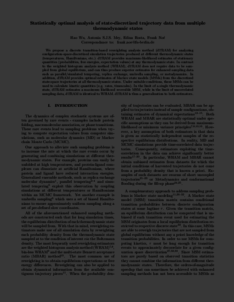Repository: Freie Universität Berlin, Math Department

# Statistically optimal analysis of state-discretized trajectory data from multiple thermodynamic states

Wu, H. and Mey, A.S.J.S. and Rosta, E. and Noé, F. (2014) Statistically optimal analysis of state-discretized trajectory data from multiple thermodynamic states. J. Chem. Phys., 141 (21). p. 214106.Preview

2MB

Official URL: http://dx.doi.org/10.1063/1.4902240

## Abstract

We propose a discrete transition-based reweighting analysis method (dTRAM) for analyzing configuration-space-discretized simulation trajectories produced at different thermodynamic states (temperatures, Hamiltonians, etc.) dTRAM provides maximum-likelihood estimates of stationary quantities (probabilities, free energies, expectation values) at any thermodynamic state. In contrast to the weighted histogram analysis method (WHAM), dTRAM does not require data to be sampled from global equilibrium, and can thus produce superior estimates for enhanced sampling data such as parallel/simulated tempering, replica exchange, umbrella sampling, or metadynamics. In addition, dTRAM provides optimal estimates of Markov state models (MSMs) from the discretized state-space trajectories at all thermodynamic states. Under suitable conditions, these MSMs can be used to calculate kinetic quantities (e.g. rates, timescales). In the limit of a single thermodynamic state, dTRAM estimates a maximum likelihood reversible MSM, while in the limit of uncorrelated sampling data, dTRAM is identical to WHAM. dTRAM is thus a generalization to both estimators.

Item Type: Article Physical Sciences Department of Mathematics and Computer Science > Institute of Mathematics > Comp. Molecular Biology 1460 BioComp Admin 11 Nov 2014 18:11 30 Nov 2017 15:13

Repository Staff Only: item control page